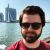# Discussion on: What's the difference between double equals (==) and triple equals (===) in JavaScript?Mike Ekkel

Hey Shane! So in their core they do the same: check equality (does left equal right). However, the way they do so differs.

The double equals (==) is an abstract equality check. What this means is that either value’s type can be converted to another type during comparison. So `0 == ‘0’` evaluates to `true`.

The triple equals (===) is a strict equality check. What this means is that it requires both values to be of the same type. So `0 == ‘0’` evaluates to `false` because type `number` is not the same as type `string`.